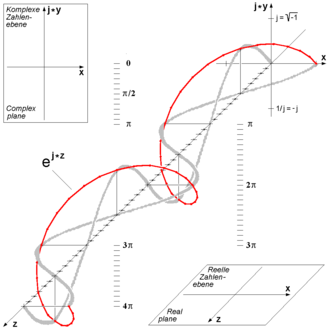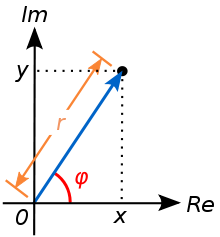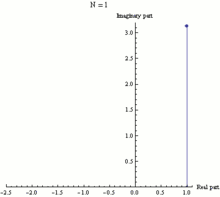# Euler's formula

﻿
Euler's formula
 Part of a series of articles on The mathematical constant eApplications in: compound interest · Euler's identity & Euler's formula  · half-lives & exponential growth/decay People John Napier  · Leonhard Euler

Euler's formula, named after Leonhard Euler, is a mathematical formula in complex analysis that establishes the deep relationship between the trigonometric functions and the complex exponential function. Euler's formula states that, for any real number x,$e^{ix} = \cos x + i\sin x \$

where e is the base of the natural logarithm, i is the imaginary unit, and cos and sin are the trigonometric functions cosine and sine respectively, with the argument x given in radians. This complex exponential function is sometimes called cis(x). The formula is still valid if x is a complex number, and so some authors refer to the more general complex version as Euler's formula.

Richard Feynman called Euler's formula "our jewel" and "one of the most remarkable, almost astounding, formulas in all of mathematics."

## History

It was Johann Bernoulli who noted that$\frac{1}{1+x^2}=\frac{1}{2} \left(\frac{1}{1-ix}+\frac{1}{1+ix} \right) \ .$

And since$\int \frac{dx}{1+ax}=\frac{1}{a}\ln(1+ax)+C \ ,$

the above equation tells us something about complex logarithms. Bernoulli, however, did not evaluate the integral. His correspondence with Euler (who also knew the above equation) shows that he didn't fully understand logarithms. Euler also suggested that the complex logarithms can have infinitely many values.

Meanwhile, Roger Cotes, in 1714, discovered that$\ln(\cos x + i\sin x)=ix \$

(where "ln" means natural logarithm, i.e. log with base e). We now know that the above equation is true modulo integer multiples of i, but Cotes missed the fact that a complex logarithm can have infinitely many values which owes to the periodicity of the trigonometric functions.

It was Euler (presumably around 1740) who turned his attention to the exponential function instead of logarithms, and obtained the correct formula now named after him. It was published in 1748, and his proof was based on the infinite series of both sides being equal. Neither of these men saw the geometrical interpretation of the formula: the view of complex numbers as points in the complex plane arose only some 50 years later (see Caspar Wessel).

## Applications in complex number theoryThis formula can be interpreted as saying that the function eix traces out the unit circle in the complex number plane as x ranges through the real numbers. Here, x is the angle that a line connecting the origin with a point on the unit circle makes with the positive real axis, measured counter clockwise and in radians.

The original proof is based on the Taylor series expansions of the exponential function ez (where z is a complex number) and of sin x and cos x for real numbers x (see below). In fact, the same proof shows that Euler's formula is even valid for all complex numbers z.

A point in the complex plane can be represented by a complex number written in cartesian coordinates. Euler's formula provides a means of conversion between cartesian coordinates and polar coordinates. The polar form simplifies the mathematics when used in multiplication or powers of complex numbers. Any complex number z = x + iy can be written as$z = x + iy = |z| (\cos \phi + i\sin \phi ) = r e^{i \phi} \$$\bar{z} = x - iy = |z| (\cos \phi - i\sin \phi ) = r e^{-i \phi} \$

where$x = \mathrm{Re}\{z\} \,$ the real part$y = \mathrm{Im}\{z\} \,$ the imaginary part$r = |z| = \sqrt{x^2+y^2}$ the magnitude of z$\phi = \arg z = \,$ atan2(y, x) .$\phi \,$ is the argument of z—i.e., the angle between the x axis and the vector z measured counterclockwise and in radians—which is defined up to addition of 2π. Many texts write tan-1(y/x) instead of atan2(y,x) but this needs adjustment when x ≤ 0.

Now, taking this derived formula, we can use Euler's formula to define the logarithm of a complex number. To do this, we also use the definition of the logarithm (as the inverse operator of exponentiation) that$a = e^{\ln (a)} \$

and that$e^a e^b = e^{a + b} \$

both valid for any complex numbers a and b.

Therefore, one can write:$z = |z| e^{i \phi} = e^{\ln |z|} e^{i \phi} = e^{\ln |z| + i \phi} \$

for any z ≠ 0. Taking the logarithm of both sides shows that:$\ln z= \ln |z| + i \phi \ .$

and in fact this can be used as the definition for the complex logarithm. The logarithm of a complex number is thus a multi-valued function, because ϕ is multi-valued.

Finally, the other exponential law$(e^a)^k = e^{a k} \ ,$

which can be seen to hold for all integers k, together with Euler's formula, implies several trigonometric identities as well as de Moivre's formula.

## Relationship to trigonometry

Euler's formula provides a powerful connection between analysis and trigonometry, and provides an interpretation of the sine and cosine functions as weighted sums of the exponential function:$\cos x = \mathrm{Re}\{e^{ix}\} ={e^{ix} + e^{-ix} \over 2}$$\sin x = \mathrm{Im}\{e^{ix}\} ={e^{ix} - e^{-ix} \over 2i} \ .$

The two equations above can be derived by adding or subtracting Euler's formulas:$e^{ix} = \cos x + i \sin x \;$$e^{-ix} = \cos(- x) + i \sin(- x) = \cos x - i \sin x \;$

and solving for either cosine or sine.

These formulas can even serve as the definition of the trigonometric functions for complex arguments x. For example, letting x = iy, we have:$\cos(iy) = {e^{-y} + e^{y} \over 2} = \cosh(y)$$\sin(iy) = {e^{-y} - e^{y} \over 2i} = -{1 \over i} {e^{y} - e^{-y} \over 2} = i\sinh(y) \ .$

Complex exponentials can simplify trigonometry, because they are easier to manipulate than their sinusoidal components. One technique is simply to convert sinusoids into equivalent expressions in terms of exponentials. After the manipulations, the simplified result is still real-valued. For example:\begin{align} \cos x\cdot \cos y & = \frac{(e^{ix}+e^{-ix})}{2} \cdot \frac{(e^{iy}+e^{-iy})}{2} \\ & = \frac{1}{2}\cdot \frac{e^{i(x+y)}+e^{i(x-y)}+e^{i(-x+y)}+e^{i(-x-y)}}{2} \\ & = \frac{1}{2} \left[ \underbrace{ \frac{e^{i(x+y)} + e^{-i(x+y)}}{2} }_{\cos(x+y)} + \underbrace{ \frac{e^{i(x-y)} + e^{-i(x-y)}}{2} }_{\cos(x-y)} \right] \ . \end{align}

Another technique is to represent the sinusoids in terms of the real part of a more complex expression, and perform the manipulations on the complex expression. For example:\begin{align} \cos(nx) & = \mathrm{Re} \{\ e^{inx}\ \} = \mathrm{Re} \{\ e^{i(n-1)x}\cdot e^{ix}\ \} \\ & = \mathrm{Re} \{\ e^{i(n-1)x}\cdot (e^{ix} + e^{-ix} - e^{-ix})\ \} \\ & = \mathrm{Re} \{\ e^{i(n-1)x}\cdot \underbrace{(e^{ix} + e^{-ix})}_{2\cos(x)} - e^{i(n-2)x}\ \} \\ & = \cos[(n-1)x]\cdot 2 \cos(x) - \cos[(n-2)x] \ . \end{align}

This formula is used for recursive generation of cos(nx) for integer values of n and arbitrary x (in radians).

## Other applications

In differential equations, the function eix is often used to simplify derivations, even if the final answer is a real function involving sine and cosine. The reason for this is that the complex exponential is the eigenfunction of differentiation. Euler's identity is an easy consequence of Euler's formula.

In electrical engineering and other fields, signals that vary periodically over time are often described as a combination of sine and cosine functions (see Fourier analysis), and these are more conveniently expressed as the real part of exponential functions with imaginary exponents, using Euler's formula. Also, phasor analysis of circuits can include Euler's formula to represent the impedance of a capacitor or an inductor.

## Definitions of complex exponentiation

The exponential function ex for real values of x may be defined in a few different equivalent ways (see Characterizations of the exponential function). Several of these methods may be directly extended to give definitions of ez for complex values of z simply by substituting z in place of x and using the complex algebraic operations. In particular we may use either of the two following definitions which are equivalent. From a more advanced perspective, each of these definitions may be interpreted as giving the unique analytic continuation of ex to the complex plane.

### Power series definition

For complex z$e^z = 1 + \frac{z}{1!} + \frac{z^2}{2!} + \frac{z^3}{3!} + \cdots = \sum_{n=0}^{\infty} \frac{z^n}{n!} ~.$

Using the ratio test it is possible to show that this power series has an infinite radius of convergence, and so defines ez for all complex z.

### Limit definition

For complex z$e^z = \lim_{n \rightarrow \infty} \left(1+\frac{z}{n}\right)^n ~.$

## Proofs

Various proofs of the formula are possible.

### Using power series

Here is a proof of Euler's formula using power series expansions as well as basic facts about the powers of i:\begin{align} i^0 &{}= 1, \quad & i^1 &{}= i, \quad & i^2 &{}= -1, \quad & i^3 &{}= -i, \\ i^4 &={} 1, \quad & i^5 &={} i, \quad & i^6 &{}= -1, \quad & i^7 &{}= -i, \end{align}

and so on. Using now the power series definition from above we see that for real values of x\begin{align} e^{ix} &{}= 1 + ix + \frac{(ix)^2}{2!} + \frac{(ix)^3}{3!} + \frac{(ix)^4}{4!} + \frac{(ix)^5}{5!} + \frac{(ix)^6}{6!} + \frac{(ix)^7}{7!} + \frac{(ix)^8}{8!} + \cdots \\[8pt] &{}= 1 + ix - \frac{x^2}{2!} - \frac{ix^3}{3!} + \frac{x^4}{4!} + \frac{ix^5}{5!} - \frac{x^6}{6!} - \frac{ix^7}{7!} + \frac{x^8}{8!} + \cdots \\[8pt] &{}= \left( 1 - \frac{x^2}{2!} + \frac{x^4}{4!} - \frac{x^6}{6!} + \frac{x^8}{8!} - \cdots \right) + i\left( x - \frac{x^3}{3!} + \frac{x^5}{5!} - \frac{x^7}{7!} + \cdots \right) \\[8pt] &{}= \cos x + i\sin x \ . \end{align}

In the last step we have simply recognized the Taylor series for sin(x) and cos(x). The rearrangement of terms is justified because each series is absolutely convergent.

### Using the limit definitionThe black point represents a complex number in the complex plane. Its absolute value is r, the distance from the origin. Its argument is φ, its angle in radians counterclockwise from the positive real axis.The exponential function ez can be defined as the limit of (1 + z/N)N, as N approaches infinity, and thus eiπ is the limit of (1 +iπ/N)N. In this animation N takes various increasing values from 1 to 100. The computation of (1 + iπ/N)N is displayed as the combined effect of N repeated multiplications in the complex plane, with the final point being the actual value of (1 +iπ/N)N. It can be seen that as N gets larger (1 +iπ/N)N approaches a limit of −1.

Here is a proof of Euler's formula starting with the definition$e^z=\lim_{n\rightarrow\infty} \left( 1+ \frac{z}{n}\right)^n$

and geometric properties of multiplication in the complex plane. In particular, a complex number z=x+iy has an absolute value defined as$|z|=\sqrt{x^2+y^2}$ and an argument defined by arg z = atan2(x,y) (atan2 is a variant of the arctangent function). In these terms, a complex multiplication z = z1z2 is performed by adding the arguments (arg(z) = arg(z1) + arg(z2)) and multiplying the absolute values ($|z| = |z_1| \, |z_2|$). (These facts are sometimes proved using Euler's theorem itself, but can alternatively be proved directly with algebra and trigonometry.) This is the basis for a two-step proof of Euler's theorem, which first calculates the absolute value of eix, and second calculates the argument of eix.

The first step is to calculate the absolute value of eix:$|e^{ix}| = \lim_{n\rightarrow\infty} \left| 1+ \frac{ix}{n}\right|^n= \lim_{n\rightarrow\infty} \left(\sqrt{1 + \frac{x^2}{n^2}}\right)^n = \lim_{n\rightarrow\infty} \left(1 + \frac{x^2}{n^2}\right)^{n/2}$

To evaluate this, there is an approximation:$(1+\delta)^m \approx 1+ \delta m$

for real numbers δ very close to 0. (This approximation is justified by the binomial theorem or by using calculus.) As n gets larger and larger, x2/n2 gets very close to zero, so we can use this approximation:$|e^{ix}| = \lim_{n\rightarrow\infty} \left(1 + \frac{x^2}{n^2}\right)^{n/2} = \lim_{n\rightarrow\infty} \left(1 + \frac{x^2}{n^2} \, \frac{n}{2} \right) = 1 + \lim_{n\rightarrow\infty} \frac{x^2}{2n} = 1$

The second step is to calculate the argument of eix. Because arg(z) = arg(z1) + arg(z2) (as mentioned above), it follows that$\arg (z^n) = n\times \arg(z)$. Therefore,$\arg (e^{ix}) = \arg \left( \lim_{n\rightarrow\infty} \left( 1+ \frac{ix}{n}\right)^n \right) = \lim_{n\rightarrow\infty} \left( n\times \arg \left(1 +\frac{ix}{n}\right)\right)$

There is an approximation:$\arg(1+i\delta) \approx \delta$

for real numbers δ very close to 0. (This approximation is related to the small-angle approximation in trigonometry,$\tan \theta \approx \theta$ when θ is small and measured in radians. It can be derived using either geometry or calculus.) Therefore,$\arg (e^{ix}) = \lim_{n\rightarrow\infty} \left( n\times \arg \left(1 +\frac{ix}{n}\right)\right)= \lim_{n\rightarrow\infty} \left( n\times \frac{x}{n} \right) = x$

Combining these two steps, eix is the complex number whose absolute value is 1 and whose argument is x. In other words, it is the point on the unit circle of the complex plane which is x radians counterclockwise from the positive real axis. This point has coordinates (cos x,sin x) in the complex plane. Therefore, eix = cos x + isin x.

### Using calculus

A well-known property of the real exponential function ex is that it equals its own derivative:$\frac{d}{dx} e^x = e^x \$ (where x is a real number).

The complex-valued exponential function also satisfies the same differential equation:$\frac{d}{dz} e^z = e^z \$ (where z is a complex number).

Therefore, by the chain rule:$\frac{d}{dx} e^{ix} = i e^{ix} \ .$

Then define the function$f(x) = (\cos x - i \sin x) \cdot e^{ix} \ .$

Because the product rule holds for complex valued functions of a real variable for the same reason as in the real case, the derivative of ƒ(x) according to the product rule is:\begin{align} \frac{d}{dx}f(x) &= (\cos x - i\sin x)\cdot\frac{d}{dx}e^{ix} + \frac{d}{dx}(\cos x - i\sin x)\cdot e^{ix} \\ &= (\cos x - i\sin x)(i e^{ix}) + (-\sin x - i\cos x)\cdot e^{ix} \\ &= (i\cos x + \sin x - \sin x - i\cos x)\cdot e^{ix} \\ &= 0 \ . \end{align}

Therefore, ƒ(x) must be a constant function in x. Because ƒ(0) = 1 by inspection, ƒ(x) = 1, giving$1 = (\cos x - i \sin x) \cdot e^{ix} \ .$

Multiplying both sides by cos x + i sin x, we obtain\begin{align} \cos x + i \sin x &= (\cos x + i \sin x)(\cos x - i \sin x) \cdot e^{ix} \\ &= (\cos^2 x -(i \sin x)^2) \cdot e^{ix} = (\cos^2 x + \sin^2 x) \cdot e^{ix} = e^{ix} \ . \end{align}

### Using differential equations

Here is another proof using calculus. The starting assumption is the same as in the previous proof:$\frac{d}{dx} e^{ix} = i e^{ix} \ .$

Define a new function ƒ(x) of the real variable x as$f(x) = \cos x + i \sin x \ .$

Then we may check that\begin{align} \frac{d}{dx}f(x) &= -\sin x + i \cos x \\ &= i f(x) \ . \end{align}

Thus ƒ(x) and eix satisfy the same first-order ordinary differential equation (here the complex values are considered as points in the plane ℝ2). Note also that both functions are equal to 1 at x = 0, then by the uniqueness of solutions to ordinary differential equations they must be equal everywhere (see Picard–Lindelöf theorem and note the comments concerning global uniqueness in the proof section there).

Wikimedia Foundation. 2010.

### Look at other dictionaries:

• Euler–Maclaurin formula — In mathematics, the Euler–Maclaurin formula provides a powerful connection between integrals (see calculus) and sums. It can be used to approximate integrals by finite sums, or conversely to evaluate finite sums and infinite series using… …   Wikipedia

• Euler's formula — 1. Math. the theorem that ei x = cos(x) + i sin(x). 2. Mech. a formula for determining the maximum load that can be applied to a given column without causing it to buckle. [1945 50; named after L. EULER] * * * Either of two important mathematical …   Universalium

• Euler's formula — noun a) Formula which links complex exponentiation with trigonometric functions: b) Formula which calculates the normal curvature of an arbitrary direction in the tangent plane in terms of the principal curvatures and and the angle which that… …   Wiktionary

• euler's formula — noun Usage: usually capitalized E : a general engineering formula relating to the strength of a long strut and obtained by mathematical analysis assuming the strut to be initially very slightly bent and neglecting the (dy/dx)² term in the… …   Useful english dictionary

• Proof of the Euler product formula for the Riemann zeta function — We will prove that the following formula holds::egin{align} zeta(s) = 1+frac{1}{2^s}+frac{1}{3^s}+frac{1}{4^s}+frac{1}{5^s}+ cdots = prod {p} frac{1}{1 p^{ s end{align}where zeta; denotes the Riemann zeta function and the product extends over… …   Wikipedia

• Fórmula de Euler-Maclaurin — En matemáticas, la fórmula de Euler Maclaurin relaciona a integrales con series. Esta fórmula puede ser usada para aproximar integrales por sumas finitas o, de forma inversa, para evaluar series (finitas o infnitas) resolviendo integrales. La… …   Wikipedia Español

• Fórmula de Euler — La fórmula o relación de Euler, atribuida a Leonhard Euler, establece que: para todo número real x. Aquí, e es la base del logaritmo natural, i es la unidad imaginaria, sin x y cos x son funciones trigonométricas. O bien: siendo z la… …   Wikipedia Español

• Euler's identity — [ 300px|thumb|right|The exponential function e z can be defined as the limit of nowrap|(1 + z / N ) N , as N approaches infinity, and thus e iπ is the limit of nowrap|(1 + iπ/N ) N . In this animation N takes various increasing values from 1 to… …   Wikipedia

• Euler's continued fraction formula — In the analytic theory of continued fractions, Euler s continued fraction formula is an identity connecting a certain very general infinite series with an infinite continued fraction. First published in 1748, it was at first regarded as a simple… …   Wikipedia

• Euler characteristic — In mathematics, and more specifically in algebraic topology and polyhedral combinatorics, the Euler characteristic is a topological invariant, a number that describes one aspect of a topological space s shape or structure. It is commonly denoted… …   Wikipedia